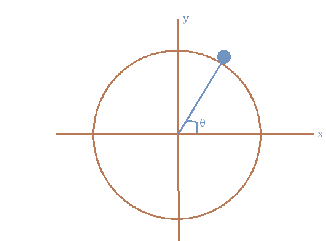# Particle on a hoop

Gold Member
Homework Statement:
Particle on a hoop - Finding the height that particle falls
Relevant Equations:
LagrangianThere is a particle with mass $m$ sliding from the hoop with radius R. Its asked to find the height of the hoop which the particles falls. Now I did the hard part (I guess) and find the Lagrangian of the system. Which is given as

$$-mR\dot{\theta}^2 + mg\sin\theta = \lambda$$
and
$$\ddot{\theta} = -\frac{g}{R}\cos\theta$$

but I cannot find the height which it falls. I have thought that when it falls from the hoop the constrain force will be ##0## (i.e, ##\lambda = 0##). But nothing seems to be working. Any ideas ?

Last edited:

Homework Helper
Gold Member
https://math.stackexchange.com/questions/1347302/particle-on-a-hemisphere-lagrange

But the solution does not make sense to me. Especially this part

\begin{equation}
\lambda=2mR\frac{g}{R}\cos\theta + mg\cos\theta = mg(3\cos\theta-2)
\end{equation}

This is clearly equal to ##3mg\cos\theta## so I don't know where that ##-2## is coming from.

Important Note: The definition of theta is different in my question and the answer given above.
The solution at stackexchange has an error. The integration constant, ##c##, in the solution is not zero. Instead, ##c = 2g/R##. This is noted in a footnote at the end of the solution. This correction accounts for the -2 in the answer for ##\lambda##.

•BvU
Gold Member
The solution at stackexchange has an error. The integration constant, ##c##, in the solution is not zero. Instead, ##c = 2g/R##. This is noted in a footnote at the end of the solution. This correction accounts for the -2 in the answer for ##\lambda##.
So how can I solve the problem.

Homework Helper
Gold Member
So how can I solve the problem.
Which parts of the solution at stackexchange are you having difficulty with?

Does the particle have a radius, or is it a point? I guess if it were point-like you wouldn't really care to use the Lagrangian formulation, so I'll just assume you're dealing with a rolling disc of radius, say, ##r##.

Use generalised coordinates ##\mathbf{q} = (\rho, \varphi, \xi)##, where ##\rho## & ##\varphi## are the plane polar coordinates of the centre of the disc and ##\xi## the angle by which the disc has turned about its centre. Introduce the holonomic constraint ##f(\mathbf{q}) = \rho - (R + r) = 0## and the non-holonomic constraint ##g(\dot{\mathbf{q}}) = (R+r)\dot{\varphi} - r\dot{\xi} = 0##. Now, write the Lagrangian$$\mathcal{L}(\mathbf{q}, \dot{\mathbf{q}}) = \frac{1}{2}m (\dot{\rho}^2 + \rho^2 \dot{\varphi}^2) + \frac{1}{2} I \dot{\xi}^2 - mg\rho \cos{\varphi}$$Lagrange's equations read\begin{align*} \frac{d}{dt} \left( \frac{\partial \mathcal{L}}{\partial \dot{\rho}} \right) - \frac{\partial \mathcal{L}}{\partial \rho} - a \frac{\partial f}{\partial \rho} - b \frac{\partial f}{\partial \dot{\rho}} &= 0 \implies m\ddot{\rho} - m \rho \dot{\varphi}^2 + mg\cos{\varphi} - a = 0 \\ \\ \frac{d}{dt} \left( \frac{\partial \mathcal{L}}{\partial \dot{\varphi}} \right) - \frac{\partial \mathcal{L}}{\partial \varphi} - a \frac{\partial f}{\partial \varphi} - b \frac{\partial f}{\partial \dot{\varphi}} &= 0 \implies m\rho^2 \ddot{\varphi} + 2m \rho \dot{\rho} \dot{\varphi} - mg\rho \sin{\varphi}-b(R+r) = 0 \\ \\ \frac{d}{dt} \left( \frac{\partial \mathcal{L}}{\partial \dot{\xi}} \right) - \frac{\partial \mathcal{L}}{\partial \xi} - a \frac{\partial f}{\partial \xi} - b \frac{\partial f}{\partial \dot{\xi}} &= 0 \implies I \ddot{\xi} + br = 0 \end{align*}Can you see how to eliminate ##b## and solve for the parameter ##a##?

Last edited by a moderator:
Gold Member
Which parts of the solution at stackexchange are you having difficulty with?
I am just looking for my own solution.
Homework Statement:: Particle on a hoop - Finding the height that particle falls
Relevant Equations:: Lagrangian

but I cannot find the height which it falls.
Does the particle have a radius, or is it a point?
Its a point particle

Its a point particle

In that case I guess you can just take ##r = I = b= 0##, ignore the third Lagrange equation and also ignore the second constraint.

Gold Member
In that case I guess you can just take ##r = I = 0## ignore the third Lagrange equation, and also ignore the second constraint.
I have already written the lagrangian. I just need to find the height it falls. How can I find it from the given equations that I have already written.

Okay, you have written ##-mR\dot{\theta}^2 + mg\sin{\theta} =\lambda## and ##\ddot{\theta} = -g/R \cos{\theta}##. You should differentiate the first equation with respect to time,$$-2mR \dot{\theta} \ddot{\theta} + mg \dot{\theta}\cos{\theta} = \frac{d\lambda}{d\theta} \dot{\theta}$$Now cancel the ##\dot{\theta}##'s and replace the ##\ddot{\theta}##,$$2mg\cos{\theta} + mg\cos{\theta} = 3mg\cos{\theta} = \frac{d\lambda}{d\theta}$$Solve with initial condition ##\lambda(\pi/2) = mg##, corresponding to the normal force at top of the hill.

Last edited by a moderator:
Mentor
I have already written the lagrangian. I just need to find the height it falls. How can I find it from the given equations that I have already written.
@Arman777 -- You have been getting excellent help in this thread so far, but you continue to show no work or effort.

This thread is locked for Moderation. Please send me a PM with your work so far so we can reopen the thread. Thank you.

•etotheipi
Mentor
OP says he has solved the problem and thanks you all for the hints and tips. thread will remain closed.